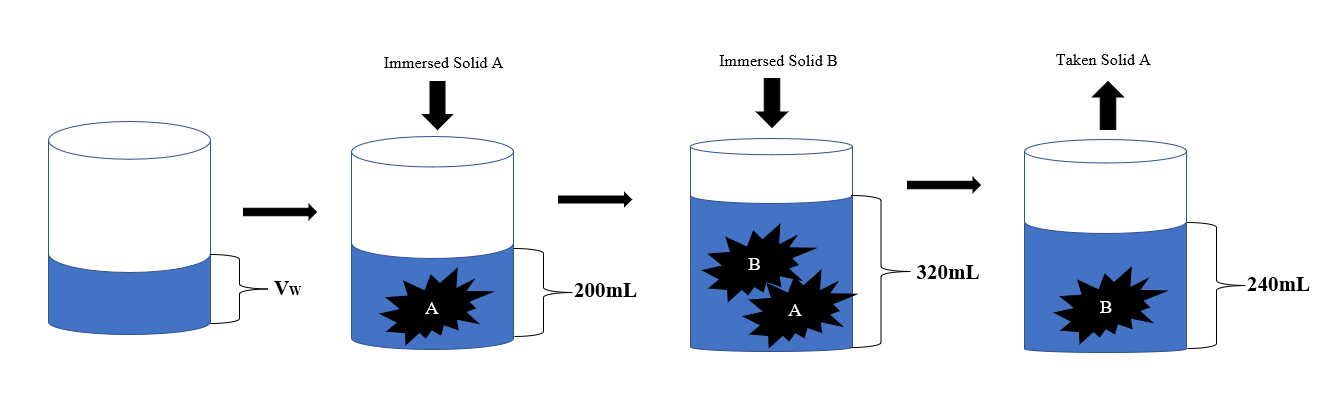# Problem of the day! (Mathematics)

in #ocd9 months ago

Irregular solid A is immersed in a graduated cylinder filled with water. The water level is at the 200 mL mark. Then, irregular solid B is immersed in the same cylinder and the water level rises to 320mL. If solid A is taken out of the cylinder, the water level will become 240mL. Which solid has the greater than volume, and how much greater over the other solid?

Visual Illustration:Solution:
Based from the illustration above
200mL = Vw + VA
320 mL = Vw + VA + VB
240 mL = Vw + VB

Where:

Vw = Volume of water inside the graduated cylinder
VA = Volume Irregular Solid A
VB = Volume Irregular Solid B

so,

200mL = Vw + VA (eq. 1)

320 mL = Vw + VA + VB (eq. 2)

240 mL = Vw + VB (eq. 3)

from eq. 1

VA = 200mL – VW (eq.4)

from eq. 3

VB = 240mL – VW (eq.5)

Substitute eq. 4 and eq. 5 to eq. 3

320 mL = Vw + (200mL – Vw) + (240mL – Vw)
320mL - 200mL – 240mL = Vw – Vw – Vw
-120mL = - Vw
Vw = 120mL

substitute the value of Vw to eq. 4 and eq. 5

VA = 200mL – 120mL = 80mL
VB = 240mL – 120mL = 120mL

Therefore;

VB is 40mL greater than VA (answer)